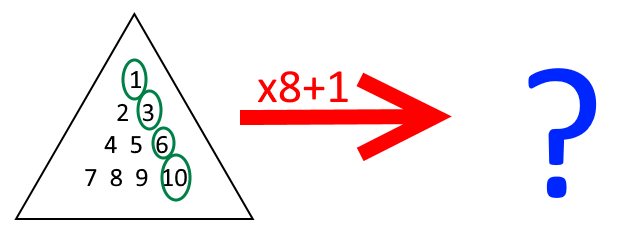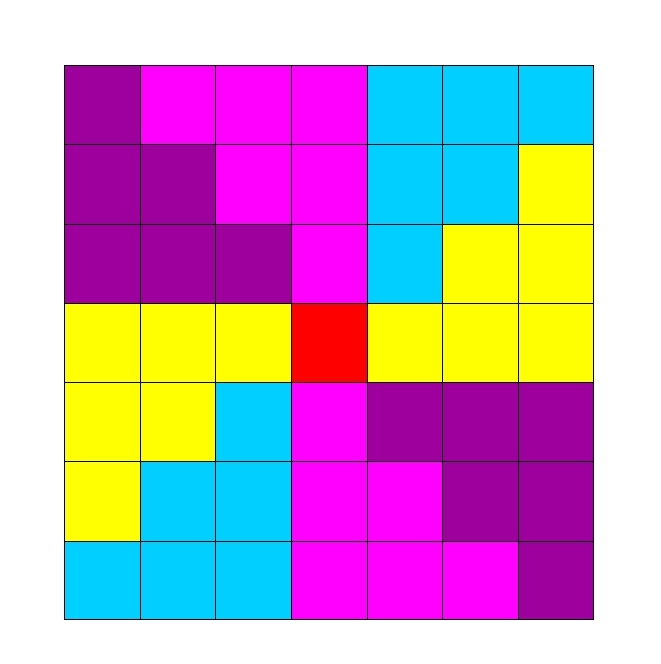#### You may also like### Proximity

We are given a regular icosahedron having three red vertices. Show that it has a vertex that has at least two red neighbours.### Kite in a Square

Can you make sense of the three methods to work out what fraction of the total area is shaded?

# Iff

##### Age 14 to 18Challenge Level

Alison has been playing with numbers again. She started by choosing a triangular number, multiplied it by 8, and added 1. She noticed something interesting about her results...Try a few examples. Can you make a conjecture?

Once you've made a conjecture of your own, click below to see what Alison noticed:

"If $T$ is a triangular number, $8T+1$ is a square number."

Can you prove the conjecture?

You might like to have a look at this Scrambled Proof and see if you can rearrange it into the original order.

Claire thought that she could use a picture to prove this conjecture. Can you use her picture to create another proof to show that the conjecture is true?I wonder if there are any integers $k$ where $8k+1$ is a square number but $k$ is not a triangular number...

Can you prove that if $8k+1$ is a square number, $k$ must be a triangular number?

Here is a Scrambled Proof for this conjecture.

Can you use your theorem to devise a quick way to check whether the following numbers are triangular numbers?

• 6214
• 3655
• 7626
• 8656

The title of this problem, "Iff", is sometimes used by mathematicians as shorthand for "If and Only If", which can also be represented by the double implication arrow $\Longleftrightarrow$. To explore the difference between "If", "Only if" and "Iff", try the problem Iffy Logic.